# Molecular Orbitals of The Allyl Cation, Allyl Radical, and Allyl Anion

Last updated: November 24th, 2020 |

How To Draw The Molecular Orbitals of The Allyl Cation, Allyl Radical And Allyl Anion

Drawing the molecular orbitals of a pi system like allyl (3 conjugated p-orbitals) is a bit like construction: build the house (orbitals) first, and fill it with people (electrons) second.

## 1. Quick Review: Molecular Orbitals For A Simple Pi Bond

In the last post, we showed how to build a molecular orbital (MO) diagram for a typical C-C pi bond.

We saw that:

• The number of molecular orbitals equalled the number of contributing atomic orbitals. The overlap of two atomic (p) orbitals gave rise to two molecular (pi, or π ) orbitals
• The lowest-energy molecular orbital had all the phases in the contributing p-orbitals aligned the same way. In other words, there were no nodes between the p-orbitals.
• Energy increases with the number of nodes (just like for standing waves). The highest-energy molecular orbital had a single node, where the p orbitals changed phase between each other.

The molecular orbitals for a pi bond look like this: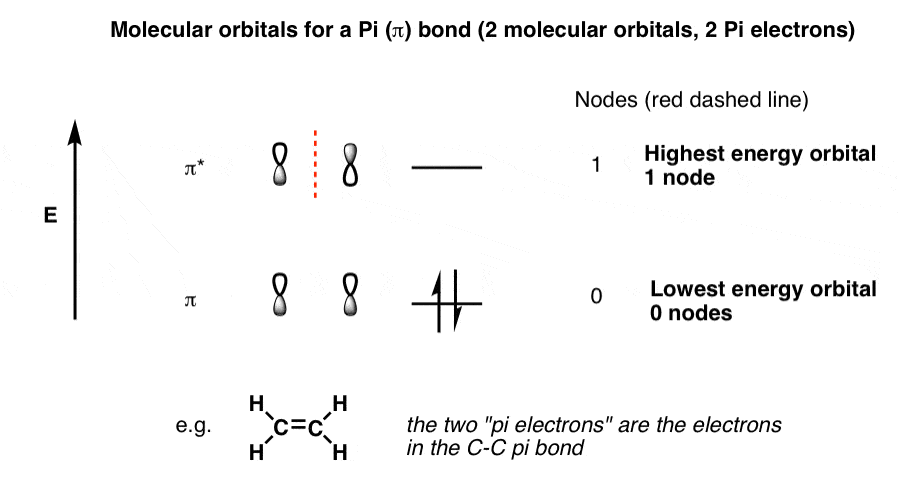Drawing out the molecular orbitals for a single pi bond seems simple enough. But what happens to the molecular orbital diagram if we add a third contributing p-orbital? Or if we have an adjacent double bond, contributing 2 further p-orbitals, giving us 4 conjugated p orbitals in total? Understanding what happens to the molecular orbitals will allow us to understand their energies, and (as we’ll see later) their reactivity.

## 2. Generalizing The Rules For Building Up Pi Molecular Orbitals

We can use the lessons learned during building up the molecular orbitals of the pi bond to generalize toward building up larger (linear) pi systems containing N molecular orbitals. [note 1]

• For a system of N atomic obitals we will have N molecular orbitals
• the lowest-energy orbital will always have zero nodes (all phase-aligned)
• The next-highest energy level up will have one node
• the level after that, two nodes,
• and so on, up to the highest energy level, which will have N-1 nodes (all of alternate phase)

Two key lessons, really. First, the number of p orbitals tells you the number of pi orbitals. Second, the lowest and highest energy orbitals are the easiest to draw: the lowest energy one will have phases all aligned, and the highest energy one will have phases all alternating. We’ll be using this generalization a lot.

So let’s take this to the next level. Three molecular orbitals. We call this the allyl system. Let’s go!

## 3. The Allyl Cation, Allyl Radical, and Allyl Anion

You’ve heard of methyl, ethyl, propyl, and butyl; our name for the three carbon unit H2C=CH-CH2  is “allyl“. Allyl alcohol, for example, is H2C=CH-CH2OH .

By now you’ve likely encountered the allyl cation, the allyl radical, or the allyl anion?  Maybe you’ve learned that the allyl cation is a “resonance-stabilized” carbocation, or the allyl radical is a “resonance stabilized” radical?In each of these “pi systems” the allyl carbon has an available p-orbital that is conjugated with the adjacent pi bond. Another way of saying the same thing is that the allyl carbon is in resonance with the pi bond, such that we can draw resonance structures of each of these molecules. [We won’t do that again here: we covered it two posts ago, in this post on  conjugation and resonance – if you need a refresher,  go back and read it to re-familiarize yourself with conjugation, resonance, and orbital overlap. ]

Since the allyl system has three p-orbitals that are in conjugation, how many Pi molecular orbitals will it have?

Three. (N=3)

Next question. What will those three pi molecular orbitals look like? Say you’re asked the following question:

“Draw the molecular orbitals of the allyl cation, the allyl radical, and the allyl anion.”

(a common enough exam question, believe it or not!).

How would you approach that?

The allyl cation, radical, and anion all utilize the same framework of molecular orbitals. They only differ in the number of pi electrons they possess (2, 3, and 4, respectively).

Knowing that, we can divide this problem into two stages.

• First, draw the orbitals themselves.
• Second, populate the orbitals with the appropriate number of pi electrons.

Let’s do this.

## 4. The Molecular Orbitals For The Allyl System (N=3)

Draw the lowest-energy and highest-energy orbitals first

We’ll start by drawing the orbitals. The two easiest to draw are the lowest-energy and highest-energy orbitals, so let’s do those first.

• The lowest-energy molecular orbital will have complete alignment of the phases in the p-orbitals, and zero nodes between them. Let’s call this “π1“, the lowest-energy pi molecular orbital. [We could alternatively have drawn the “shaded” phases all on top: it amounts to the same thing].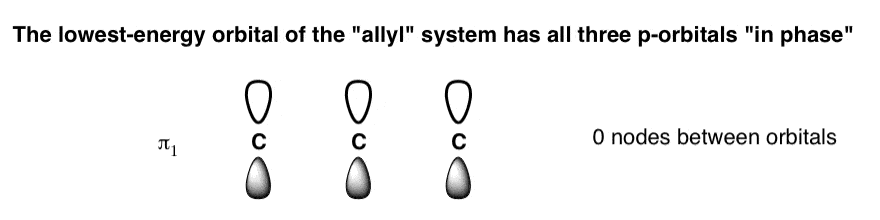• The highest-energy molecular orbital is also easy to draw: all the phases will alternate, giving it (N-1) = 2 nodes. We can call this “π3“, the highest-energy pi orbital of the three. The nodes are marked with red lines.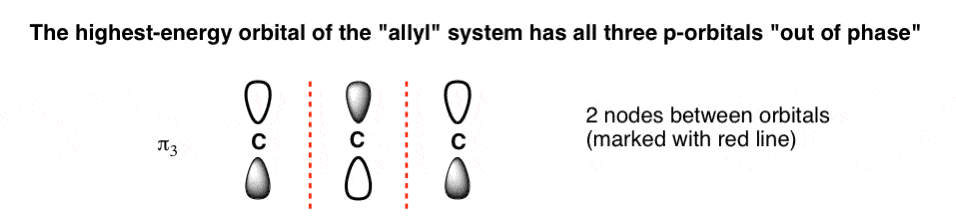## 5. The Tricky Middle Orbital Of The Allyl System

What about the middle orbital, π2 ? It should have one node, and be intermediate in energy between π1 and π3

Where to put that single node? If you’re like I was, your first temptation for drawing the orbitals will likely be to put a node between one of the carbons, giving orbitals that look something like this: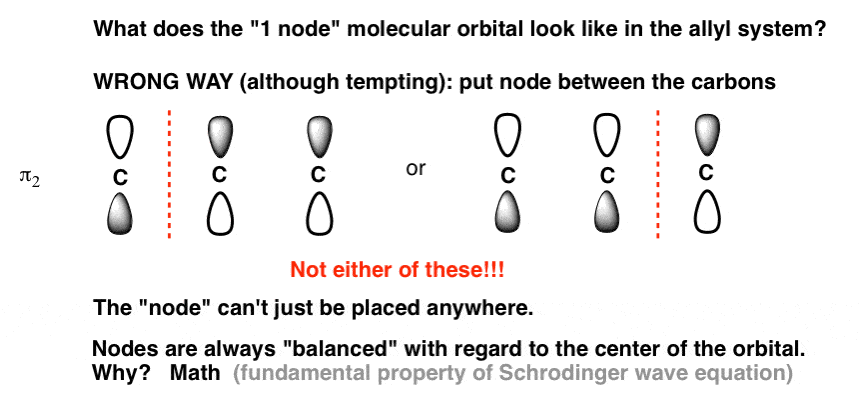It’s actually incorrect!

Why? That’s a good question, and the answer isn’t obvious. It turns out that because of the way the math works in the Schrodinger equation, the nodes are always “balanced” with respect to the centre of the orbital. The node can’t just be placed anywhere; when there is one node, it must be in the centre. [By the way, this is a general feature of MO’s where the number of contributing orbitals is odd, e.g. N=3, N=5, N=7… the first node will be on the central carbon.]

The Node Goes In The Middle!

That means that the node is directly on that middle carbon.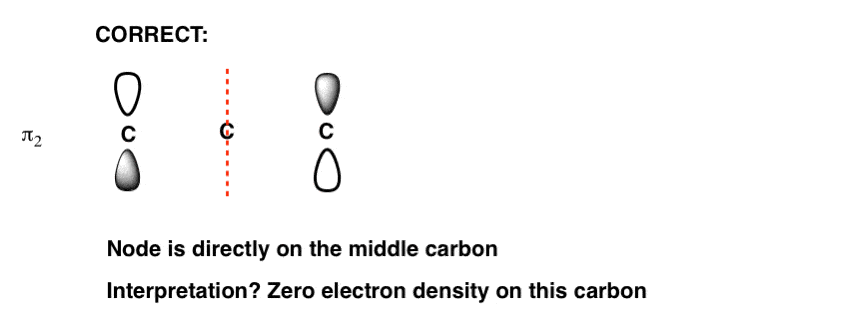Weird, right? What does that actually mean? It means that in that molecular orbital there is zero electron density on that central carbon. We don’t need to go into further detail for our purposes, but I’m including a note where it gives us some clues about reactivity. [note 2]

Now, if we arrange these orbitals together by energy (most stable at bottom) we get the following orbital diagram for the allyl system.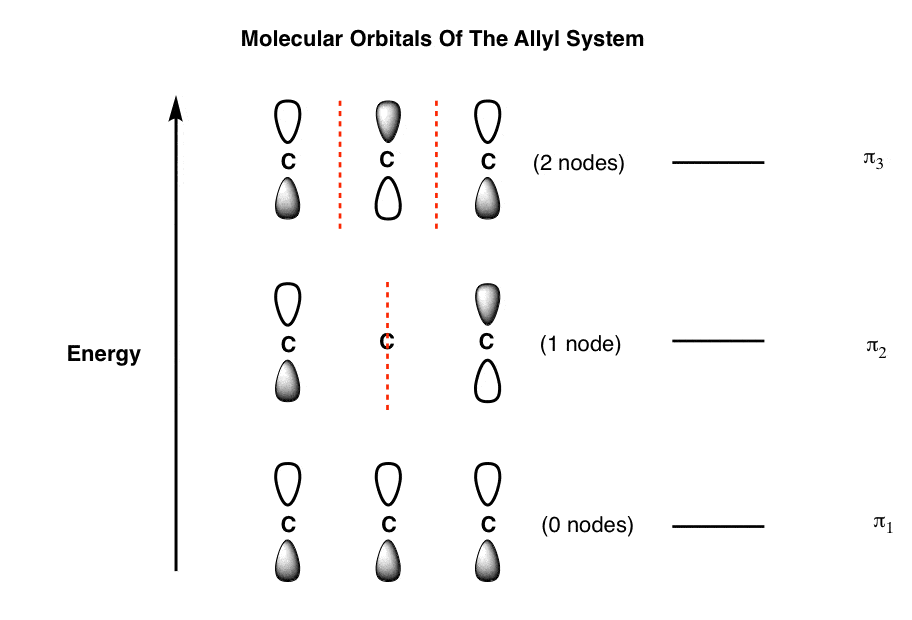This is just the framework for the orbitals in the allyl system. It’s not specific to the allyl cation, radical, or anion yet, because those three species differ in the number of Pi electrons they possess.

Now, let’s populate the orbitals with electrons.

## 6. Molecular Orbitals of The Allyl Cation (2 pi electrons)

In the allyl cation we have 2 pi electrons in total: in the resonance form below, we see two electrons in the pi bond, and zero on the allyl carbon.

When we populate our allyl orbitals with two electrons, building from the ground floor up, we get the following: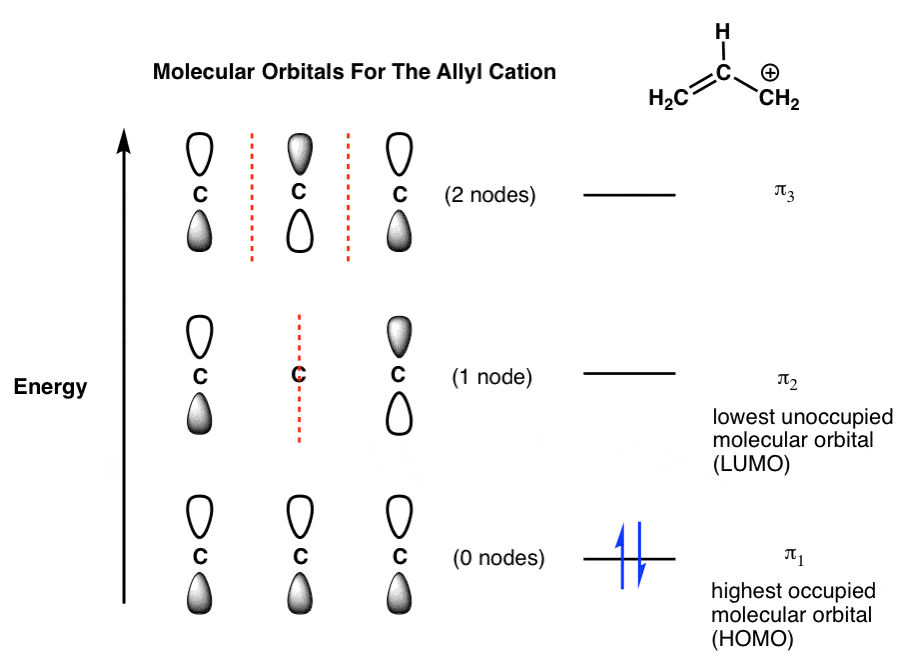Note that the “highest occupied” molecular orbital of the allyl cation is π1 – the lowest-energy orbital in the system. The “lowest unoccupied” molecular orbital is π.

We generally abbreviate the terms “highest occupied molecular orbital”  as HOMO and “lowest-unoccupied molecular orbital ” as LUMO. They are often called the “frontier” molecular orbitals  and are where most of the action happens in reactions, as we’ll see in future posts.

## 7. Molecular Orbitals of the Allyl Radical (3 pi electrons)

What about the allyl radical? Here we have 3 pi electrons: two in the pi bond, and a third one in that half-filled p orbital.

Where do we put that third electron? In the next-highest orbital, of course. Into π2 it goes!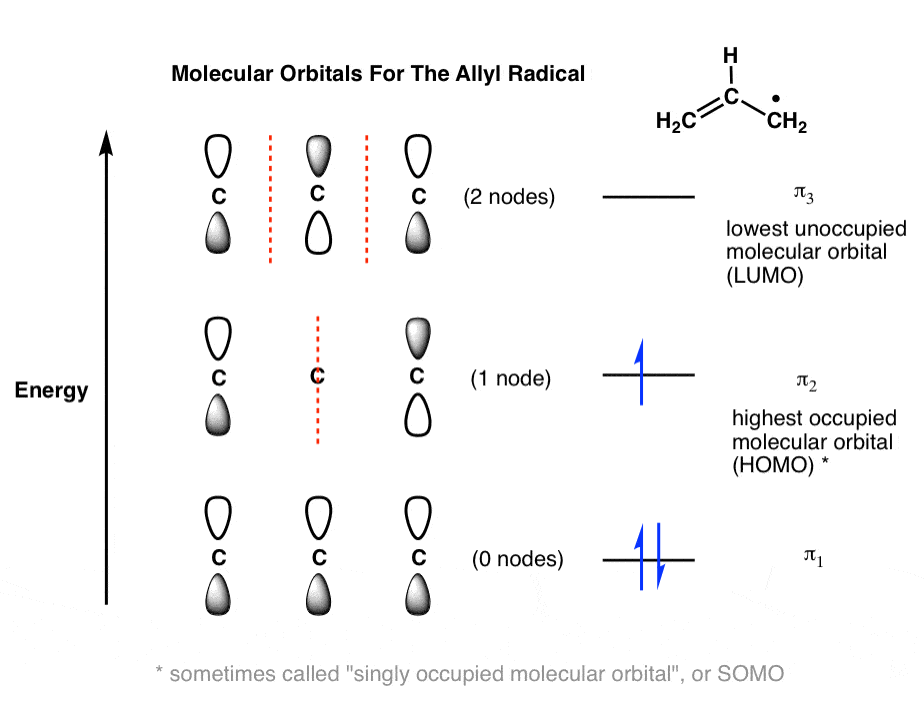That gives us a half-filled π2, which is our new “HOMO”, and πnow becomes our “LUMO”. [I don’t want to add to the confusion, but sometimes half-filled molecular orbitals like this are referred to as a “singly occupied molecular orbital”, or SOMO. Not super-important at this stage].

## 8. Molecular Orbitals of the Allyl Anion (4 pi electrons)

What about the allyl anion? Here we have 4 pi electrons: two in the pi bond, and two more as a lone pair on the terminal carbon.  This fills up π.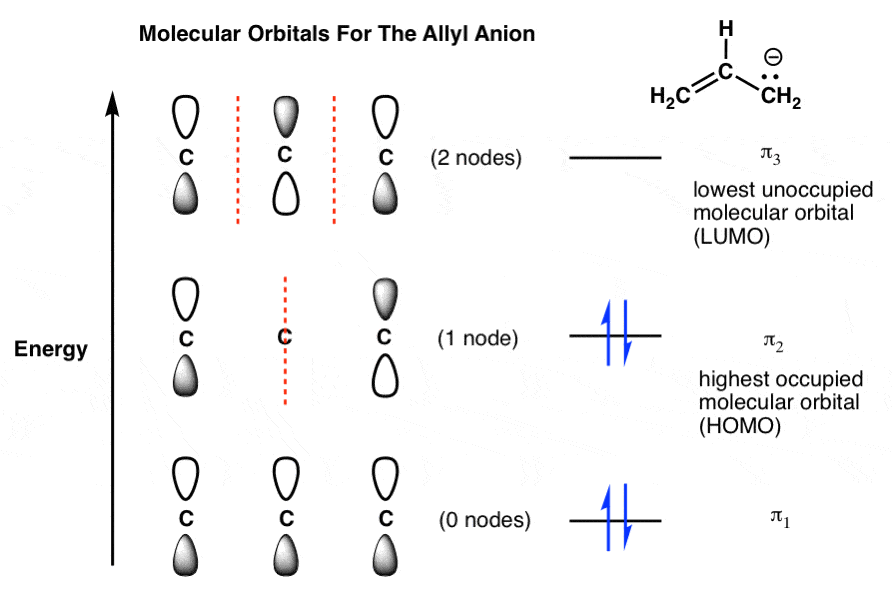Our HOMO remains πand our LUMO remains π3 .

This satisfactorily shows the molecular orbitals for the allyl cation, radical, and anion.

## 9. Conclusion: The Allyl System

Here, we’ve shown how to extend the general principles of molecular orbitals we learned in building up the molecular orbitals of a pi bond (N=2)  toward the allyl system (N=3). The key points are as follows:

• N atomic p orbitals give rise to N pi molecular orbitals
• The Nth molecular orbital will have N-1 nodes. (e.g. the 3rd MO in the allyl system has (3–1 = 2) nodes.
• When asked to draw molecular orbitals, a good rule of thumb is to start with the lowest-energy and highest-energy molecular orbitals. The lowest-energy molecular orbital has zero nodes (all p orbitals in phase) and the highest-energy molecular orbital will have (N-1) nodes (all p orbitals out of phase).
• Don’t add electrons to the system until you’ve drawn out all the molecular orbitals first.

In the next post, let’s look at the molecular orbitals for the butadienyl system (N=4).

## Notes

Note 1. The rule of thumb that for “the Nth molecular orbital will have N-1 nodes” applies best to linear molecules. Cyclic molecules (such as benzene) follow the same general principles, but due to symmetry will have “nodal planes”. For example the lowest energy MO of benzene has zero nodes, but the next-highest energy level of benzene is “doubly degenerate” meaning that there are two ways to draw a single nodal plane. More here. We’ll cover this in a separate post.

[An advanced note: one consequence of the node being in the centre of π2 is that  the radical has zero electron density in the middle carbon (C-2). This is consistent with everything we’ve ever observed about the reactivity of allyl radicals – they react at the termini (C-1 or C-3), and never the middle carbon (C-2). ]

Final note. Here’s a final (advanced) footnote, that only the extremely curious should look at. It’s showing how the 3 molecular orbitals of the allyl system are formed through interaction of two Pi molecular orbitals with a p orbital. This results in lowering of the energy of the  π orbital (resulting in a stabilization of energy E) and raising of the energy of the  π* orbital. The energy of the p orbital remains unchanged; intermediate in energy between bonding and antibonding, it is referred to as a “nonbonding” molecular orbital.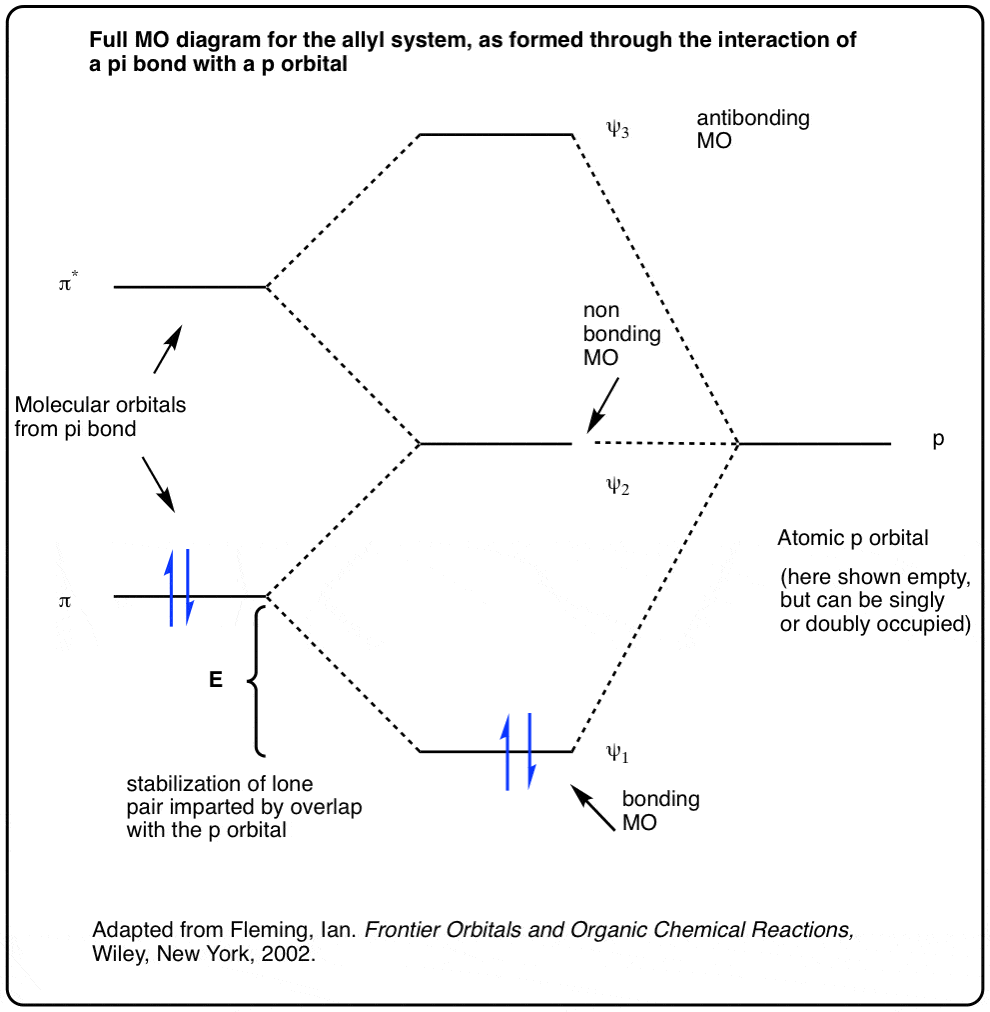Adapted from Ian Fleming’s excellent “Frontier Orbitals and Organic Chemical Reactions”.

## Comment section

### 10 thoughts on “Molecular Orbitals of The Allyl Cation, Allyl Radical, and Allyl Anion”

1.Anustup says:

Thanks a lot Sir for a really helpful post ….. easy to understand yet difficult to find out in ordinary textbooks.

2.Peter says:

Really great explanation!

1.James Ashenhurst says:

3.Yashika says:

4.Yashika says:

Thank you so much for your quick response. You’re right, I did mean allene and I’m quite familiar with it’s structure. What I was wondering was whether the molecular orbitals formed from the atomic orbitals will exist independently and whether they will also be perpendicular to each other.

1.James Ashenhurst says:

Yes, they will exist independently and yes, they will be perpendicular to each other. In essence they operate like two independent pi bonds.

5.Rahul Krishnakumar says:

Thank you very much Sir for these lovely explanations!

6.shubham says:

thankyou for giving such detailed answer and explaining in a simple way :)

This site uses Akismet to reduce spam. Learn how your comment data is processed.